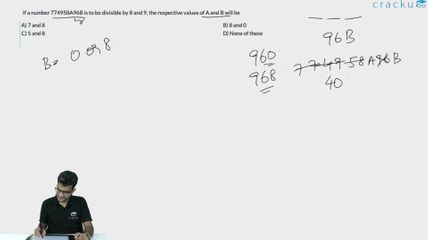Question 6

# If a number 774958A96B is to be divisible by 8 and 9, the respective values of A and B will be

Solution

According to the divisible rule of 9, the sum of all digits should be divisible by 9.
i.e. 55+A+B = 9k
So sum can be either 63 or 72.
For 63, A+B should be 8.
In given options, option B has values of A and B whose sum is 8 and by putting them we are having a number which divisible by both 9 and 8.

### View Video Solution